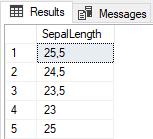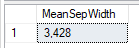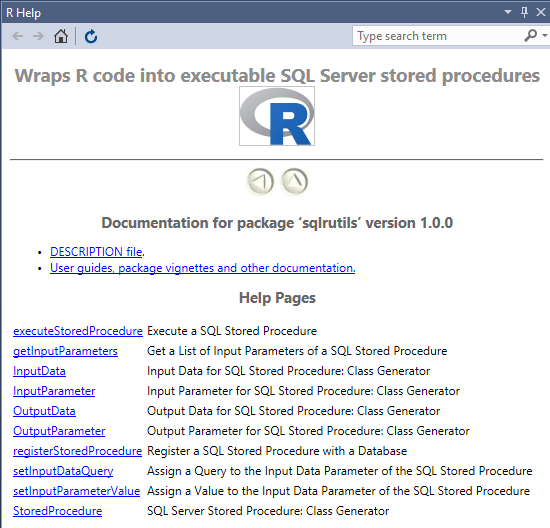# Creating R Stored Procedures in SQL Server 2016 Using sqlrutils

In the Microsoft R Server forum the other day was a question about inputs to SQL Server R stored procedures, or rather about “strange” parameter naming, when using the sqlrutils package. I got intrigued by the question and started playing around with researching it, and this blog-post is the result. Oh, and this blog-post would never have seen the light of the day, if it hadn’t been for the original poster of the question: JD Long, thanks JD!

So, what is SQL Server R stored procedures and what is sqlrutils?

Before we answer the question above, let’s do a quick recap of `sp_execute_external_script`.

## `sp_execute_external_script`

The procedure `sp_execute_external_script` is an extended stored procedure, and it allows us to execute R scripts (and in SQL 2017 also Python) from inside SQL Server. You use it by passing it the script you want to execute as well as various parameters. Let’s assume you have some R code looking like so:

``````# set a variable
multiplier <- 5

#get the iris data set as a data frame
iris_dataset <- iris

# grab the setosa species
setosa <- iris[iris\$Species == 'setosa',]

# calculate mean of the Sepal.Width for setosa
menSepWidth <- mean(setosa\$Sepal.Width)

# use the multiplier to do some "stuff
iris_dataset\$Sepal.Length <- iris_dataset\$Sepal.Length * multiplier

# look at the resulting dataset
View(iris_dataset\$Sepal.Length)

# print out the mean
print(menSepWidth)
``````

Code Snippet 1: R Script

As you see in Code Snippet 1, we:

• Set the value of the `multiplier` variable.
• Reads in the `iris` data into a data frame: `iris_dataset`.
• Create a new data frame for the `setosa` species.
• Calculate the `mean` of the `Sepal.Width` for `setosa` and assigns that to a variable: `menSepWidth`.
• Multiply the `Sepal.Length` property with the `multiplier` variable.
• Finally output the calculated property, and print out the `menSepWidth` variable.

Yes, yes, I know - the code is very, very simplistic, but it will still get the points across.

NOTE: If you haven’t heard about the `iris` dataset before, you can read more about it here.

To use this script in SQL Server with `sp_execute_external_script`, you would write something like this:

``````DECLARE @out_val float;

exec sp_execute_external_script
@language = N'R',
@script = N'
iris_dataset <- iris

setosa <- iris[iris\$Species == "setosa",]

menSepWidth <- mean(setosa\$Sepal.Width)

iris_dataset\$Sepal.Length <- iris_dataset\$Sepal.Length * multiplier
OutputDataSet <- data.frame(iris_dataset\$Sepal.Length)
',
@params = N'@multiplier float, @menSepWidth float OUTPUT',
@multiplier = 5,
@menSepWidth = @out_val OUTPUT
WITH RESULT SETS ((SepalLength float));

SELECT @out_val AS MeanSepWidth
``````

Code Snippet 2: The iris Script in SQL Server

As you see from Code Snippet 2, the R script is passed in to the `@script` parameter of `sp_execute_external_script`. The actual script looks almost identical to what is in Code Snippet 1, except for instead of the `View(iris_dataset\$Sepal.Length)`, we use `OutputDataSet`. The `OutputDataSet` variable is a well known variable in SQL Server R Services, and it is the default variable that will contain the data to be returned to SQL Server as a result-set upon completion of the stored procedure call. The layout of the result-set is defined in the `WITH RESULT SETS` statement, and in Code Snippet 2 above we are interested in one column, of type `float` and we name it `SepalLength`.

The parameters we use are defined in the `@params` parameter, followed by the parameters themselves. In this case `@multiplier` which we set to 5, and `@menSepWidth` which is `OUTPUT`. If you have used `sp_executesql` the parameter syntax should be familiar to you. We assign the (at the top of the script) declared `@out_val` variable to the `@menSepWidth` output parameter, and at the end of the script we `SELECT` it out.

When running Code Snippet 2, the output will be like in the two figures below:Figure 1: Result-set

Figure 1 shows the output from `OutputDataSet`, the calculated `Sepal.Length` column.Figure 2: Output Parameter

In Figure 2 we see the output parameter; `@menSepWidth`.

This is nice, being able to execute `sp_execute_external_script`, and run our R scripts in SQL Server. However how do I operationalize this, I can’t really be running these scripts every time I want to “crunch” some data?! Well, the answer to that is to wrap the code we see in Code Snippet 2 in a stored procedure, which would take the necessary parameters, and then execute `sp_execute_external_script` inside the procedure.

To make it easier to create wrapping procedures, Microsoft has an R package to help with this: sqlrutils.

## sqlrutils

The sqlrutils package allows R users to, from inside an R IDE of choice, put their R scripts into a T-SQL stored procedure, register that stored procedure with a database, and run the stored procedure from an R development environment.

Let us see what the package does. To do that run from inside an R IDE, I am using R Tools for Visual Studio 2017 (RTVS), `help(package="sqlrutils")`.

NOTE: Before you run the `help` statement above, you may have to run: `library(sqlrutils)`, to load the package.

When running `help(package=“sqlrutils”), the httpd help server starts and you should see something like so:Figure 3: Output from Help

In Figure 3 you see the various function the package exposes. Now, let’s see what we can do with it.

### R Function

It is considered best practices to rewrite your R script as a function, and pass necessary parameters to the function. So we take our script and turn it into a function:

``````irisFunc <- function(multiplier) {

iris_dataset <- iris

setosa <- iris[iris\$Species == 'setosa',]

menSepWidth <- mean(setosa\$Sepal.Width)

iris_dataset\$Sepal.Length <- iris_dataset\$Sepal.Length * multiplier

sepLength <- data.frame(iris_dataset\$Sepal.Length)

retList <- list(sepLengthData = sepLength,
menSepWidthValue = menSepWidth)

return(retList)
}
``````

Code Snippet 3: Create Function

In Code Snippet 3 we create the function and we:

• pass in the `multiplier` parameter
• declare the `menSepWidth` variable inside the function
• assign `iris_dataset\$Sepal.Length` to a data frame variable
• create a named list, containing the data frame as well as `menSepWidth` variable
• return the list

The function will eventually be part of the script inside `sp_execute_external_script`, but before we go there we should define the required parameters for the procedure.

### Define Parameters

In this example we have three parameters / objects:

• the `multiplier` input parameter
• the `menSepWidth` output parameter
• the output dataset

When looking at the output from the `help` as in Figure 3, we see some functions that looks like doing what we want to do:

• `InputParameter`
• `OutputData`
• `OutputParameter`

So to create our parameters we write some code like in Code Snippet 4:

``````# if the sqlrutils package is not loaded yet:
# library("sqlrutils")

inParam <- InputParameter("multiplier", "numeric")
outParam <- OutputParameter("menSepWidthValue", "numeric")
outputData <- OutputData("sepLengthData")
``````

Code Snippet 4: Parameter Creation

Notice how the output dataset, and the output parameter are named as the names in the list. This is important as we will see in a little bit. You can have multiple parameters (input/output), but only one output data set. If you have multiple parameters you define them as in Code Snippet 4.

Now when the parameters are defined, we can create the stored procedure.

### Procedure Creation

To create a stored procedure we use the `StoredProcedure` class generator, and the signature of `StoredProcedure` looks like so:

``````StoredProcedure(func,
spName,
...,
filePath = NULL,
dbName = NULL,
connectionString = NULL,
batchSeparator = "GO")
``````

Code Snippet 5: Signature for StoredProcedure Class Generator

The arguments in the signature are:

• `func` - the function name, of the function we want wrapped.
• `spName` - name for the generated stored procedure
• `...` - optional input and output parameters (including output dataset)
• `filePath` - optional path to where to create and save a .sql source file.
• `dbName` - optional name specifying the database to use in the script file.
• `connectionString` - optional connection string.
• `batchSeparator` - what batch separator to use.

So, let’s see what we should do to create a stored procedure based on what we have done so far (the code snippet below includes the parameter definitions from *Code Snippet 4):

``````inParam <- InputParameter("multiplier", "numeric")
outParam <- OutputParameter("menSepWidthValue", "numeric")
outputData <- OutputData("sepLengthData")

irisProc <- StoredProcedure(irisFunc,
"pr_IrisProc",
inParam,
outputData,
outParam,
filePath = "C:\\Temp"
)
``````

Code Snippet 6: Generate Stored Procedure from R Function

In Code Snippet 6 we:

• define the function to use is the function assigned to the `irisFunc` variable from Code Snippet 3.
• say that we want the created procedure to be named `pr_IrisProc`.
• define the input and output parameters though the variables we have created. If you have defined more parameters (as we spoke about above), you add them to the `StoredProcedure` call.
• set the file path where we want the source files to be created.

We skip the rest of the arguments, and when we run the code in Code Snippet 6, the source file looks like so:

``````IF (OBJECT_ID('pr_IrisProc') IS NOT NULL)
DROP PROCEDURE pr_IrisProc
GO
CREATE PROCEDURE pr_IrisProc
@parallel_outer bit = 0,
@multiplier_outer float,
@menSepWidthValue_outer float output
AS
BEGIN TRY
exec sp_execute_external_script
@language = N'R',
@script = N'
irisFunc <- function (multiplier)
{
iris_dataset <- iris
setosa <- iris[iris\$Species == "setosa", ]
menSepWidth <- mean(setosa\$Sepal.Width)
iris_dataset\$Sepal.Length <- iris_dataset\$Sepal.Length *
multiplier
sepLength <- data.frame(iris_dataset\$Sepal.Length)
retList <- list(sepLengthData = sepLength, menSepWidthValue = menSepWidth)
return(retList)
}
result <- irisFunc(multiplier = multiplier)
if (is.list(result)) {
OutputDataSet <- result\$sepLengthData
menSepWidthValue <- result\$menSepWidthValue
} else stop("the R function must return a list")
',
@parallel = @parallel_outer,
@params = N'@multiplier float, @menSepWidthValue float output',
@multiplier = @multiplier_outer,
@menSepWidthValue = @menSepWidthValue_outer output
END TRY
BEGIN CATCH
THROW;
END CATCH;
GO
``````

Code Snippet 7: Generated Stored Procedure

That looks OK, a couple of things to point out:

• Parameter names are appended with _outer. This is what JD Long asked about; where comes that from? It seems to be the convention to do that, to separate the parameters for `sp_execute_external_script` from the parameters for the outer procedure. Hence the _outer in the name.
• What’s this `@parallel_outer` parameter, we did not define it anywhere? In `sp_execute_external_script` you have the ability to define that you wish the execution of the R script should happen in parallel, and `@parallel` is an existing parameter on `sp_execute_external_script`. So by default, this parameter is always created (and defaulted to 0).
• We then see the function definition as part of the `@script` parameter, together with generated code to execute and retrieve the results. This is where it is important that you return a named list from the function, especially if you have more than one output parameter (including the output dataset). The names in the list should be the same as you set in your parameter definitions.

You can now take the source file and deploy the procedure to a database of your choice. Then you execute the procedure as so:

``````DECLARE @out float;
EXEC pr_IrisProc @parallel_outer = 0,
@multiplier_outer = 5,
@menSepWidthValue_outer = @out OUT;
SELECT @out;
``````

Code Snippet 8: Execution of Generated Procedure

When executing, you should get the same result as you see in Figure 1 and Figure 2.

### Deploying from R

Above we deployed the created procedure by running the script from SQL Server Management Studio, on the database we wanted to deploy to. You can also deploy straight from R, by using `registerStoredProcedure`. The signature of `registerStoredProcedure` looks like in Code Snippet 8:

``````registerStoredProcedure(sqlSP,
connectionString = NULL)
``````

Code Snippet 8: Signature of registerStoredProcedure

We see in Code Snippet 8 how `registerStoredProcedure` takes the generated stored procedure object (created by `StoredProcedure`), and an optional connection string. It is optional as the connection string can be defined in `StoredProcedure`.

If we want to deploy to SQL Server directly the code looks like so:

``````conStr <- paste0("Driver={ODBC Driver 13 for SQL Server};Server=.;",

registerStoredProcedure(irisProc, conStr)
``````

Code Snippet 9: Deploying from R

In Code Snippet 9, we continue from Code Snippet 6, and we have created the stored procedure object `irisProc`. We create a connection string, and pass `irisProc` and the connection string to `registerStoredProcedure`. After executing the code in Code Snippet 9, you should see the procedure in the database.

As you know, by using `sp_execute_external_script`, we can now in SQL Server 2016 and 2017 execute R scripts (and in SQL 2017 also Python) from inside SQL Server. `sp_execute_external_script` is a SQL Server extended stored procedure and you pass in the script to execute as well as parameter info to the procedure.

## ~ Finally

If you have comments, questions etc., please comment on this post or ping me.